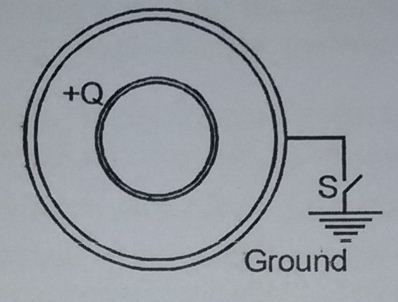# Shells Losing Energy !An arrangement of two concentric conducting spherical shells charged initially such that the inner shell (radius = $a$) carries a net charge of $+Q$ while outer shell (radius $b$) carries net charge $0$. If the switch "$S$" is closed, how much electrostatic potential energy would the system lose?

×